#Quality Digest

Featured Product
This Week in Quality Digest Live
Statistics Features
William A. Levinson
People can draw the wrong conclusions due to survivor, survey, and bad news bias.
Donald J. Wheeler
Does your approach do what you need?
Paul Laughlin
Correlation vs. causality
Donald J. Wheeler
In spite of what everyone says to the contrary
Donald J. Wheeler
What can you say about the sampled lot?
Statistics News
Elsmar Cove is a leading forum for quality and standards compliance
InfinityQS’ quality solutions have helped cold food and beverage manufacturers around the world optimize quality and safety
User friendly graphical user interface makes the R-based statistical engine easily accessible to anyone
Collect measurements, visual defect information, simple Go/No-Go situations from any online device
Good quality is adding an average of 11 percent to organizations’ revenue growth
Ability to subscribe with single-user minimum, floating license, and no long-term commitment
A guide for practitioners and managers
Gain visibility into real-time quality data to improve manufacturing process efficiency, quality, and profits
Tool for nonstatisticians automatically generates models that glean insights from complex data setsBio

Statistics

## So What Are Skewness and Kurtosis?

### And what do they add to the story?

Published: Tuesday, September 7, 2021 - 12:03

What do the shape statistics known as skewness and kurtosis tell us about our data? Last month we saw how the average and standard deviation define the balance point and radius of gyration for our data. Once we have these two quantities the empirical rule tells us where the bulk of the data should be found. Here we look at the contributions of skewness and kurtosis.

In Statistics Summer Camp we used building blocks to create digital distributions. These digital models allowed us to see how the location and dispersion statistics work to describe the data. Here we will use the same four digital models to examine skewness and kurtosis. We use digital models because they not only provide analogs for the continuous probability models but also share the characteristics of actual histograms of data. For information on how to create these and other digital models please refer to the appendix of last month’s column.

### Four digital models

Our four digital probability models each use 200 building blocks to approximate a standardized probability model. Each block is centered as close as the measurement increment of 0.1 unit will allow to the x-value for the median of each interval containing 0.005 of the area under the standardized probability distribution.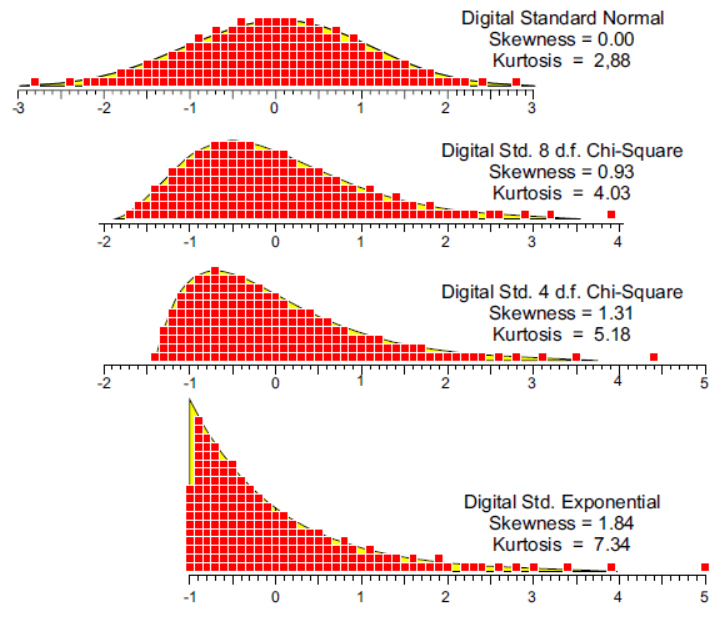Figure 1: Four digital models

In spite of their different shapes these four models have means that are essentially zero and variances that are essentially 1.00. Thus, they are equivalent in terms of their balance points and their rotational inertias. And, as we saw last month, they all have at least 95 percent of their area between –2.0 and +2.0, and they also have at least 98.5 percent of their area between –3.0 and +3.0.

### What is skewness?

More than 100 years ago Karl Pearson gave us the basic formulas for skewness and kurtosis. Letting RMSD denote the root mean squared deviation: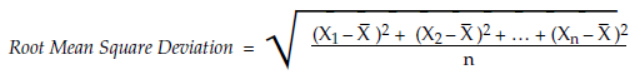the basic skewness statistic is: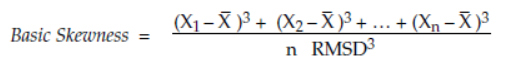The basic skewness starts with the deviation of each value from the average. These deviations are cubed and added up. This sum is divided by the number of data points, n, and then divided by the cube of the root mean square deviation. This last step standardizes this statistic and turns it into a pure number with no measurement units attached.

While your software may dress this quantity up in various ways, all of the commonly used formulas are based on the basic statistic given by Karl Pearson. For example, Excel uses the following: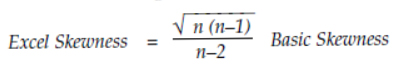For discrete standardized models like those in figure 1 the formula for the basic skewness simplifies to become approximately: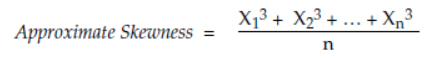Because of the symmetry of the normal distribution the cubed negative values exactly cancel out the cubed positive values, resulting in a skewness of zero. For the three skewed models the situation is slightly more complex.

When computing the skewness for the digital standardized 8 d.f. chi-square model we find that the sum of all the cubed negative values will almost cancel out the sum of the cubed positive values for those blocks between 0 and 2.0.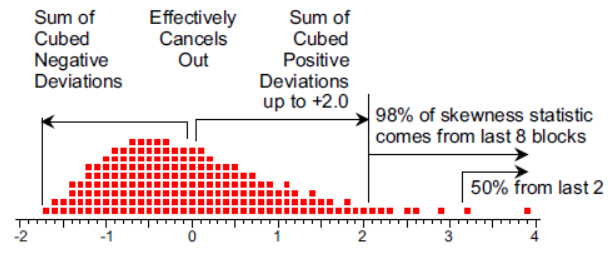Figure 2: Skewness for the digital 8 d.f. chi-square model

Thus, +2.0 is the zero-skewness balance point for this model, and the skewness statistic of 0.928 is essentially dependent upon the last 8 blocks (from 2.1 to 3.9). Moreover, half of this skewness value comes from the last two blocks at 3.2 and 3.9.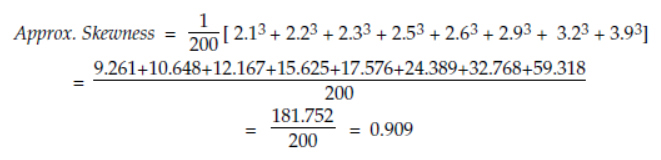(The simplified computation above of 0.909 assumes the MSD value is 1.000. Here the MSD is 0.9850 which inflates the computed skewness 2.3% to yield the computed value of 0.928.)

When computing the skewness for the digital standardized 4 d.f. chi-square model we find that the sum of all the cubed negative values will almost cancel out the sum of the cubed positive values for those blocks between 0 and 1.8.Figure 3: The 4 d.f. digital chi-square

Thus, +1.8 is the zero-skewness balance point for this model, and the skewness statistic of 1.309 is essentially dependent upon the last 11 blocks (from 1.9 to 4.4). Moreover, half of this skewness statistic comes from the last two blocks at 3.5 and 4.4.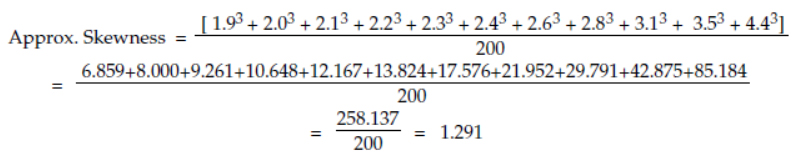When computing the skewness for the digital standardized exponential model we find that the sum of all the cubed negative values will almost cancel out the sum of the cubed positive values for those blocks between 0 and 1.7.Figure 4: The digital exponential

Thus, +1.7 is the zero-skewness balance point for this model, and the skewness statistic of 1.836 is essentially dependent upon the last 13 blocks (from 1.8 to 5.0). More than half of this skewness statistic comes from the last two blocks at 3.9 and 5.0.So we see that for skewed models, skewness is almost wholly dependent on that portion of the elongated tail that is more than 2 standard deviations away from the mean. Moreover, about half of the skewness comes from the most extreme 1 percent of the blocks in these digital models.

### What is kurtosis?

Karl Pearson’s formula for the basic kurtosis is:The basic kurtosis starts with the deviations from the average. These deviations are raised to the fourth power and added up. This sum is divided by the number of data points, n, and then divided by the root mean square deviation raised to the fourth power. This last step standardizes this statistic and turns it into a number with no measurement units attached.

Excel dresses the basic kurtosis statistic up in the following way:Unlike skewness, all 200 of the blocks contribute to the kurtosis. However, raising the deviations to the fourth power minimizes the contributions of those blocks within two standard deviations of the mean. Thus, kurtosis is essentially dependent upon the outer tails (beyond ±2.0) and is highly influenced by any points in the extreme tails (beyond ±3.0). These contributions are summarized in figure 5.

With the short but heavy tails of the digital normal model we find that 49 percent of the kurtosis depends upon the most extreme 8 blocks (beyond ±2.0) in the model.Figure 5: Kurtosis depends on the outer tails

With the longer tails of the skewed models we find the most extreme 8 or 9 blocks determine from 72 percent to 90 percent of the kurtosis value while the most extreme 2 or 3 blocks contribute from 43 percent to 76 percent of the kurtosis.

### Skewness and kurtosis in practice

While the examples above use probability models, the formulas work with these digital models in the same way that they function with histograms. So even though the formulas for the skewness and kurtosis will incorporate all of the data, both of these quantities are heavily dependent upon the most extreme 1 percent to 5 percent of those data. This dependence upon the extreme values makes these statistics highly variable in practice.

To illustrate this consider a data set consisting of the following 25 values:We are going to consider how changes in the last value (3.8 above) affect the skewness and kurtosis for the data set as a whole. The basic skewness and kurtosis values for the original 25 data are 2.000 and 8.012. Figure 6 shows how these values change as we repeatedly move the last point to the left by one-half unit.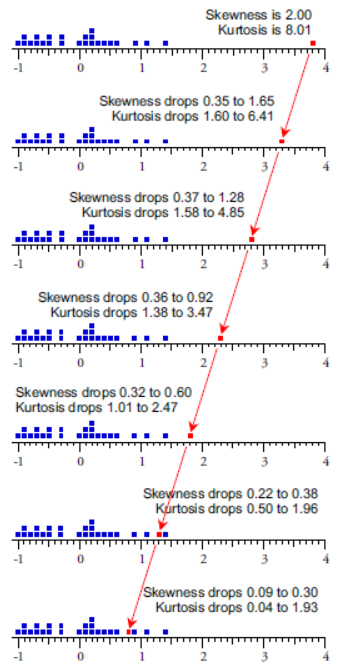Figure 6: Shape statistics depend upon the extreme value

While 24 of the 25 values remain the same, with each change in the last value the skewness and kurtosis statistics drop. As long as the point being moved is the most extreme point its value has a major impact upon the shape statistics. In the last two data sets, as the point being moved ceases to be the most extreme point, it has less and less impact upon the shape statistics. Thus, when working with data sets involving a few dozen values, the skewness and kurtosis can depend upon the location of a single value.

But what happens with larger data sets? To answer this question I generated 5,000 data sets of n = 200 observations using a standard normal probability model. This continuous probability model has a skewness parameter of zero and a kurtosis parameter of 3.00.

The skewness statistics for these 5,000 data sets averaged –0.002 and ranged from –0.68 to +0.61. 95 percent of the skewness values fell between –0.34 and +0.33. This uncertainty of plus or minus 0.33 is too much variation to allow any practical use of the skewness statistic.

The kurtosis statistics for these 5,000 data sets averaged 2.97 and ranged from 2.21 to 5.15. 95 percent of these kurtosis values fell between 2.44 and 3.77. Once again, this uncertainty of plus 0.77 and minus 0.56 is too much variation to allow any practical use of the kurtosis statistic. Neither the skewness statistic nor the kurtosis statistic will provide useful estimates for the shape parameters of any probability model. (For more on this question see “Problems With Skewness and Kurtosis, Part 2,” Quality Digest, August 1, 2011.)

### Summary

While the skewness and kurtosis formulas appear to utilize all of the data, these shape statistics are essentially functions of the most extreme 5 percent of the data, and are heavily dependent upon the most extreme 1 percent of the data. They do not have any direct connection to the overall “shape” of a histogram. Rather they attempt to measure the extremity of the extreme values. This undermines their usefulness in characterizing the data set as a whole.

Once we have characterized the location and dispersion we have essentially extracted all of the useful information that can be obtained from numerical summaries of the data.

Plots of the data in their time-order sequence and in a histogram can complement numerical summaries by revealing nonquantitative information, but additional computations beyond location and dispersion add no real value.

Finally, as seen above, skewness and kurtosis essentially ignore the central 95 percent of the data in any histogram. So you should return the favor by ignoring the skewness and kurtosis statistics provided by your software. There is simply nothing to be learned from these so-called shape statistics.

### Discuss### Donald J. Wheeler

Find out about Dr. Wheeler’s virtual seminars for 2022 at www.spcpress.com. Dr. Wheeler is a fellow of both the American Statistical Association and the American Society for Quality who has taught more than 1,000 seminars in 17 countries on six continents. He welcomes your questions; you can contact him at djwheeler@spcpress.com.